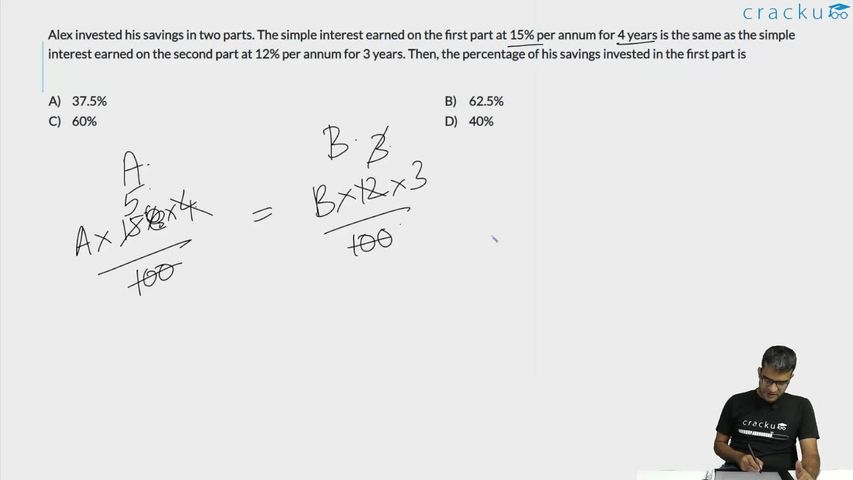Question 64

# Alex invested his savings in two parts. The simple interest earned on the first part at 15% per annum for 4 years is the same as the simple interest earned on the second part at 12% per annum for 3 years. Then, the percentage of his savings invested in the first part is

Solution

Let the savings invested in first part and second part be 'x' and 'y', respectively.

It is given,

$$\ \frac{\ x\times15\times4}{100}=\ \frac{\ y\times12\times3}{100}$$

60x = 36y

5x = 3y

Required percentage = $$\frac{x}{x+y}\times100=\frac{3}{3+5}\times100=37.5\%$$

### View Video Solution